Chapter 7. Energy and Chemistry

# Formation Reactions

### Learning Objectives

1. Define a formation reaction and be able to recognize one.
2. Use enthalpies of formation to determine the enthalpy of reaction.

Hess’s law allows us to construct new chemical reactions and predict what their enthalpies of reaction will be. This is a very useful tool because now we don’t have to measure the enthalpy changes of every possible reaction. We need measure only the enthalpy changes of certain benchmark reactions and then use these reactions to algebraically construct any possible reaction and combine the enthalpies of the benchmark reactions accordingly.

But what are the benchmark reactions? We need to have some agreed-on sets of reactions that provide the central data for any thermochemical equation.

Formation reactions are chemical reactions that form one mole of a substance from its constituent elements in their standard states. By standard states we mean as a diatomic molecule if that is how the element exists and the proper phase at normal temperatures (typically room temperature). The product is one mole of substance, which may require that coefficients on the reactant side be fractional (a change from our normal insistence that all coefficients be whole numbers). For example, the formation reaction for methane (CH4) is

C(s) + 2 H2(g) → CH4(g)

The formation reaction for carbon dioxide (CO2) is

C(s) + O2(g) → CO2(g)

In both cases, one of the elements is a diatomic molecule because that is the standard state for that particular element. The formation reaction for H2O—

2 H2(g) + O2(g) → 2 H2O(ℓ)

—is not in a standard state because the coefficient on the product is 2; for a proper formation reaction, only one mole of product is formed. Thus, we have to divide all coefficients by 2:

H2(g) + 1/2 O2(g) → H2O(ℓ)

On a molecular scale, we are using half of an oxygen molecule, which may be problematic to visualize. However, on a molar level, it implies that we are reacting only half of a mole of oxygen molecules, which should be an easy concept for us to understand.

### Example 11

Which of the following are proper formation reactions?

1. H2(g) + Cl2(g) → 2 HCl(g)
2. Si(s) + 2 F2(g) → SiF4(g)
3. CaO(s) + CO2 → CaCO3(s)

Solution

1. In this reaction, two moles of product are produced, so this is not a proper formation reaction.
2. In this reaction, one mole of a substance is produced from its elements in their standard states, so this is a proper formation reaction.
3. One mole of a substance is produced, but it is produced from two other compounds, not its elements. So this is not a proper formation reaction.

Test Yourself

Is this a proper formation reaction? Explain why or why not.

2 Fe(s) + 3 P(s) + 12 O(g) → Fe2(PO4)3(s)

Answer

This is not a proper formation reaction because oxygen is not written as a diatomic molecule.

Given the formula of any substance, you should be able to write the proper formation reaction for that substance.

### Example 12

Write formation reactions for each of the following.

1. FeO(s)
2. C2H6(g)

Solution

In both cases, there is one mole of the substance as product, and the coefficients of the reactants may have to be fractional to balance the reaction.

1. Fe(s) + 1/2 O2(g) → FeO(s)
2. 2 C(s) + 3 H2(g) → C2H6(g)

Test Yourself

Write the equation for the formation of CaCO3(s).

Answer

Ca(s) + C(s) + 3/2 O2(g) → CaCO3(s)

The enthalpy change for a formation reaction is called the enthalpy of formation The subscript f is the clue that the reaction of interest is a formation reaction. Thus, for the formation of FeO(s),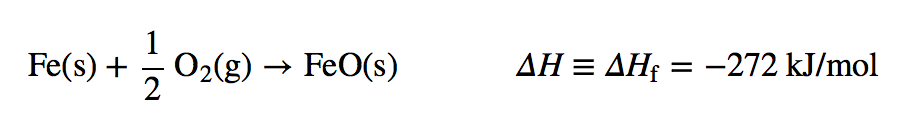Note that now we are using kJ/mol as the unit because it is understood that the enthalpy change is for one mole of substance. Note, too, by definition, that the enthalpy of formation of an element is exactly zero because making an element from an element is no change. For example,

H2(g) → H2(g) ΔHf = 0

Formation reactions and their enthalpies are important because these are the thermochemical data that are tabulated for any chemical reaction. Table 7.2 “Enthalpies of Formation for Various Substances” lists some enthalpies of formation for a variety of substances; in some cases, however, phases can be important (e.g., for H2O).

It is easy to show that any general chemical equation can be written in terms of the formation reactions of its reactants and products, some of them reversed (which means the sign must change in accordance with Hess’s law). For example, consider

2 NO2(g) → N2O4(g)

We can write it in terms of the (reverse) formation reaction of NO2 and the formation reaction of N2O4: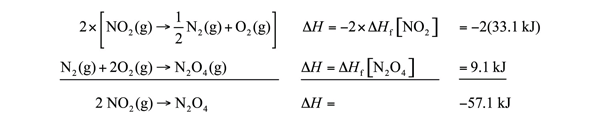We must multiply the first reaction by 2 to get the correct overall balanced equation. We are simply using Hess’s law in combining the ΔHf values of the formation reactions.

Table 7.2 Enthalpies of Formation for Various Substances

Compound ΔHf (kJ/mol) Compound ΔHf (kJ/mol) Compound ΔHf (kJ/mol) Compound ΔHf (kJ/mol)
Ag(s) 0 Ca(s) 0 Hg2Cl2(s) −265.37 NaHCO3(s) −950.81
AgBr(s) −100.37 CaCl2(s) −795.80 I2(s) 0 NaN3(s) 21.71
AgCl(s) −127.01 CaCO3(s, arag) −1,207.1 K(s) 0 Na2CO3(s) −1,130.77
Al(s) 0 CaCO3(s, calc) −1,206.9 KBr(s) −393.8 Na2O(s) −417.98
Al2O3(s) −1,675.7 Cl2(g) 0 KCl(s) −436.5 Na2SO4(s) −331.64
Ar(g) 0 Cr(s) 0 KF(s) −567.3 Ne(g) 0
Au(s) 0 Cr2O3(s) −1,134.70 KI(s) −327.9 Ni(s) 0
BaSO4(s) −1,473.19 Cs(s) 0 Li(s) 0 O2(g) 0
Br2(ℓ) 0 Cu(s) 0 LiBr(s) −351.2 O3(g) 142.67
C(s, dia) 1.897 F2(g) 0 LiCl(s) −408.27 PH3(g) 22.89
C(s, gra) 0 Fe(s) 0 LiF(s) −616.0 Pb(s) 0
CCl4(ℓ) −128.4 Fe2(SO4)3(s) −2,583.00 LiI(s) −270.4 PbCl2(s) −359.41
CH2O(g) −115.90 Fe2O3(s) −825.5 Mg(s) 0 PbO2(s) −274.47
CH3COOH(ℓ) −483.52 Ga(s) 0 MgO(s) −601.60 PbSO4(s) −919.97
CH3OH(ℓ) −238.4 HBr(g) −36.29 NH3(g) −45.94 Pt(s) 0
CH4(g) −74.87 HCl(g) −92.31 NO(g) 90.29 S(s) 0
CO(g) −110.5 HF(g) −273.30 NO2(g) 33.10 SO2(g) −296.81
CO2(g) −393.51 HI(g) 26.5 N2(g) 0 SO3(g) −395.77
C2H5OH(ℓ) −277.0 HNO2(g) −76.73 N2O(g) 82.05 SO3(ℓ) −438
C2H6(g) −83.8 HNO3(g) −134.31 N2O4(g) 9.08 Si(s) 0
C6H12(ℓ) −157.7 H2(g) 0 N2O5(g) 11.30 U(s) 0
C6H12O6(s) −1277 H2O(g) −241.8 Na(s) 0 UF6(s) −2,197.0
C6H14(ℓ) −198.7 H2O(ℓ) −285.83 NaBr(s) −361.1 UO2(s) −1,085.0
C6H5CH3(ℓ) 12.0 H2O(s) −292.72 NaCl(s) −385.9 Xe(g) 0
C6H6(ℓ) 48.95 He(g) 0 NaF(s) −576.6 Zn(s) 0
C10H8(s) 77.0 Hg(ℓ) 0 NaI(s) −287.8 ZnCl2(s) −415.05
C12H22O11(s) −2,221.2

### Example 13

Show that the reaction

Fe2O3(s) + 3 SO3(g) → Fe2(SO4)3(s)

can be written as a combination of formation reactions.

Solution

There will be three formation reactions. The one for the products will be written as a formation reaction, while the ones for the reactants will be written in reverse. Furthermore, the formation reaction for SO3 will be multiplied by 3 because there are three moles of SO3 in the balanced chemical equation. The formation reactions are as follows: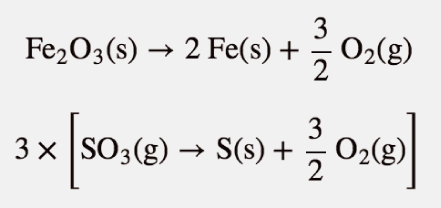2 Fe(s) + 3 S(s) + 6 O2(g) → Fe2(SO4)3(s)

When these three equations are combined and simplified, the overall reaction is

Fe2O3(s) + 3 SO3(s) → Fe2(SO4)3(s)

Test Yourself

Write the formation reactions that will yield

2 SO2(g) + O2(g) → 2 SO3(g).

Answer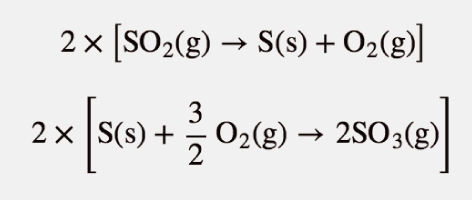Now that we have established formation reactions as the major type of thermochemical reaction we will be interested in, do we always need to write all the formation reactions when we want to determine the enthalpy change of any random chemical reaction? No. There is an easier way. You may have noticed in all our examples that we change the signs on all the enthalpies of formation of the reactants, and we don’t change the signs on the enthalpies of formation of the products. We also multiply the enthalpies of formation of any substance by its coefficient—technically, even when it is just 1. This allows us to make the following statement: the enthalpy change of any chemical reaction is equal to the sum of the enthalpies of formation of the products minus the sum of the enthalpies of formation of the reactants. In mathematical terms,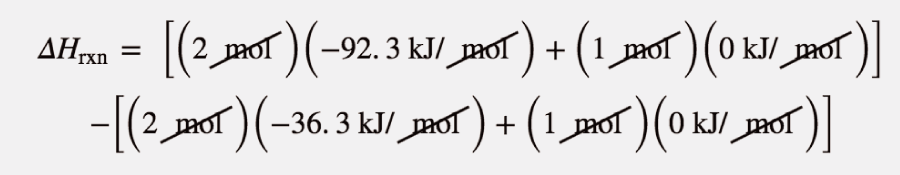where np and nr are the number of moles of products and reactants, respectively (even if they are just 1 mol), and ΔHf,p and ΔHf,r are the enthalpies of formation of the product and reactant species, respectively. This products-minus-reactants scheme is very useful in determining the enthalpy change of any chemical reaction, if the enthalpy of formation data are available. Because the mol units cancel when multiplying the amount by the enthalpy of formation, the enthalpy change of the chemical reaction has units of energy (joules or kilojoules) only.

### Example 14

Use the products-minus-reactants approach to determine the enthalpy of reaction forSolution

The enthalpies of formation are multiplied by the number of moles of each substance in the chemical equation, and the total enthalpy of formation for reactants is subtracted from the total enthalpy of formation of the products:

ΔHrxn=[(2 mol)(−92.3 kJ/ mol)+(1 mol)(0 kJ/ mol)]−[(2 mol)(−36.3 kJ/ mol)+(1 mol)(0 kJ/ mol)]

All the mol units cancel. Multiplying and combining all the values, we get

ΔHrxn = −112.0 kJ

Test Yourself

What is the enthalpy of reaction for this chemical equation?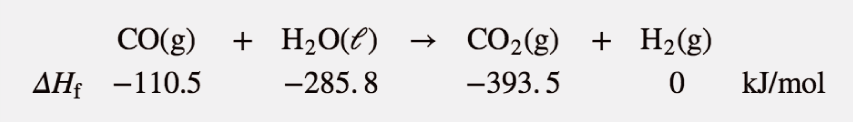Answer

+2.8 kJ

### Food and Drink App: Calories and Nutrition

Section 7.1 “Energy” mentioned the connection between the calorie unit and nutrition: the calorie is the common unit of energy used in nutrition, but we really consider the kilocalorie (spelled Calorie with a capital C). A daily diet of 2,000 Cal is actually 2,000,000 cal, or over 8,000,000 J, of energy.

Nutritionists typically generalize the Calorie content of foods by separating it into the three main food types: proteins, carbohydrates, and fats. The general rule of thumb is as follows:

If the food is It has this energy content
protein 4 Cal/g
carbohydrate 4 Cal/g
fat 9 Cal/g

This table is very useful. Assuming a 2,000 Cal daily diet, if our diet consists solely of proteins and carbohydrates, we need only about 500 g of food for sustenance—a little more than a pound. If our diet consists solely of fats, we need only about 220 g of food—less than a half pound. Of course, most of us have a mixture of proteins, carbohydrates, and fats in our diets. Water has no caloric value in the diet, so any water in the diet is calorically useless. (However, it is important for hydration; also, many forms of water in our diet are highly flavoured and sweetened, which bring other nutritional issues to bear.)

When your body works, it uses calories provided by the diet as its energy source. If we eat more calories than our body uses, we gain weight—about 1 lb of weight for every additional 3,500 Cal we ingest. Similarly, if we want to lose weight, we need to expend an extra 3,500 Cal than we ingest to lose 1 lb of weight. No fancy or fad diets are needed; maintaining an ideal body weight is a straightforward matter of thermochemistry—pure and simple.

### Key Takeaways

• A formation reaction is the formation of one mole of a substance from its constituent elements.
• Enthalpies of formation are used to determine the enthalpy change of any given reaction.

### Exercises

1. Define formation reaction and give an example.

2. Explain the importance of formation reactions in thermochemical equations.

3. Which of the following reactions is a formation reaction? If it is not a formation reaction, explain why.

a)  H2(g) + S(s) → H2S(g)

b)  2 HBr(g) + Cl2(g) → 2 HCl(g) + Br2(ℓ)

4.  Which of the following reactions is a formation reaction? If it is not a formation reaction, explain why.

a)  Fe(g) + 1/2 O2(g) → FeO(s)

b)  Hg(ℓ) + 1/2 O2(g) → HgO(s)

5.  Which of the following reactions is a formation reaction? If it is not a formation reaction, explain why.

a)  H2(g) + S(s) + 2 O2(g) → H2SO4(ℓ)

b)  C3H8(g) + 5 O2(g) → 3 CO2(g) + 4 H2O(ℓ)

6.  Which of the following reactions is a formation reaction? If it is not a formation reaction, explain why.

a)  Zn(s) + 2 HCl(aq) → ZnCl2(aq) + H2(g)

b)  2 Na(s) + C(s) + 3/2 O2(g) → Na2CO3(s)

7.  Write a proper formation reaction for each substance.

a)  H3PO4(s)

b)  Na2O(s)

c)  C3H7OH(ℓ)

8.  Write a proper formation reaction for each substance.

a)  N2O5(g)

b)  BaSO4(s)

c)  Fe(OH)3(s)

9.  Write a proper formation reaction for each substance.

a)  C12H22O11(s)

b)  Zn(NO3)2(s)

c)  Al(OH)3(s)

10.  Write a proper formation reaction for each substance.

a)  O3(g)

b)  Na2O2(s)

c)  PCl5(g)

11.  Write this reaction in terms of formation reactions.

MgCO3(s) → MgO(s) + CO2(g)

12.  Write this reaction in terms of formation reactions.

2 NO + 4 NO2 → 2 N2O5 + N2

13. Write this reaction in terms of formation reactions.

2 CuCl(s) → Cu(s) + CuCl2(s)

14.  Write this reaction in terms of formation reactions.

SiH4 + 4 F2 → SiF4 + 4 HF

15.  Determine the enthalpy change of this reaction. Data can be found in Table 7.2 “Enthalpies of Formation for Various Substances”.

CH2O(g) + O2 → CO2(g) + H2O(ℓ)

16.  Determine the enthalpy change of this reaction. Data can be found in Table 7.2 “Enthalpies of Formation for Various Substances”.

2 AgBr(s) + Cl2(g) → 2 AgCl(s) + Br2(ℓ)

17.  Determine the enthalpy change of this reaction. Data can be found in Table 7.2 “Enthalpies of Formation for Various Substances”.

Mg(s) + N2O5(g) → MgO(s) + 2NO2(g)

18.  Determine the enthalpy change of this reaction. Data can be found in Table 7.2 “Enthalpies of Formation for Various Substances”.

2C6H6(ℓ) + 15O2(g) → 12CO2(g) + 6H2O(ℓ)

Answers

1.

A formation reaction is a reaction that produces one mole of a substance from its elements. Example: C(s) + O2(g) → CO2(g)

3.

a)  formation reaction

b)  It is not the formation of a single substance, so it is not a formation reaction.

5.

a)  formation reaction

b)  It is not the formation of a single substance, so it is not a formation reaction.

7.

a)  3/2 H2(g) + P(s) + 2 O2(g) → H3PO4(s)

b)  2 Na(s) + 1/2 O2(g) → Na2O(s)

c)  3 C(s) + 1/2 O2(g) + 4 H2(g) → C3H7OH(ℓ)

9.

a)  12 C(s) + 11 H2(g) + 11/2 O2(g) → C12H22O11(s)

b)  Zn(s) + N2(g) + 3 O2(g) → Zn(NO3)2

c)  Al(s) + 3/2 O2(g) + 3/2 H2(g) → Al(OH)3(s)

11.

a)  MgCO3(s) → Mg(s) + C(s) + 3/2 O2(g)

b)  Mg(s) + 1/2 O2(g) → MgO(s)

c)  C(s) + O2(g) → CO2(g)

13.

a)  2 × [CuCl(s) → Cu(s) + 1/2 Cl2(g)]

b)  Cu(s) → Cu(s)

c)  Cu(s) + Cl2(g) → CuCl2(s)

15.

ΔH = −563.44 kJ

17.

ΔH = −546.7 kJ

## LicenseFormation Reactions by Jessie A. Key is licensed under a Creative Commons Attribution-NonCommercial-ShareAlike 4.0 International License, except where otherwise noted.